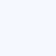By Kevin Watts (Article from RebreatherWorld.Com)

Gradient Factors for Dummies attempts to provide a user’s view of gradient factors, an Erik Baker derived method of calculating decompression schedules. The title is not meant to be derisive, but simply an indicator that this article is meant to be a primer.

## Back to Buhlmann

Everything in the gradient factor decompression algorithm revolves around Buhlmann’s tissue model. Currently this means 16 hypothetical tissue compartments (TCs) that are constantly tracked during a dive in order to determine each TC’s inert gas pressure.

As you ascend all those TCs start to release pressure (“off- gas”). The question is “How fast can you let those TCs off-gas?”

Buhlmann answered that question by coming up with an “M-value”. Basically, an M-value is a maximum pressure value (different for each depth and tissue compartment) that tells you, if you exceed that value, Buhlmann thinks you’re crazy and believes you’re about to get bent.

A natural ascent strategy, then, would be to move up in the water column until the pressure in your TCs just reaches Buhlmann’s M-value and then let your TCs off-gas a bit, rise to the next level, etc. In this strategy, you would keep going up in such a way that you never let your TCs exceed Buhlmann’s M-value.

Unfortunately, decompression illness does not exactly track Buhlmann’s M-values. More sickness occurs at and above the pressures represented by M-values and less sickness occurs when divers never reach Buhlmann’s M-values.

Gradient factors (GFs) were invented to let the diver choose how fast, and how close, their TCs get to Buhlmann’s M-values.

Gradient factors are calculated as follows: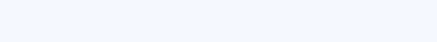### What does this formula tell us?

First, the gradient factor formula tells us that at a GF=1.0, you are at Buhlmann’s M-value. Therefore, staying at or below GF=1.0 seems important. Second, it tells us that when our tissue compartment pressure just reaches ambient pressure, then the GF=0.0.

Another ascent strategy, then, might be to shoot up to a GF=0.8 and ascend in such a way as not to exceed that value. In this way you know that your tissue compartments are never over 80% of the distance between ambient pressure and Buhlmann’s M-value. In essence, you have a 20% safety margin on Buhlmann’s M-values. Dive computers implementing GFs usually let you set two GF parameters. Moving straight to GF=0.8 and ascending in such a way that you always keep your TCs at GF=0.8 would be equivalent to setting your dive computer to 80/80.

## Erik Baker’s Strategy

Erik Baker didn’t like the idea of ascending directly to a GF close to Buhlmann’s M-Value. Instead he said, “Let’s all ascend first to a lower GF, then slowly move to higher GFs.” So, let’s say you want to first ascend to a GF=0.30 and then slowly move to reach GF=0.85 as you surface. This setting on your gradient factor computer is 30/85. In fact, my Shearwater computer uses 30/85 as it’s default setting.

### So what is happening when you use a GF setting of 30/85?

First your dive computer allows you to ascend until the pressure in your TCs first reaches a GF=0.3. This means your TC pressure is 30% of the way between ambient pressure and Buhlmann’s M-value. Then you sit there until your TCs drop enough pressure so that you can ascend to your next stop.

### How much pressure must leave your TCs before you can ascend?

Assume you hit your first stop (GF=0.3) at 110 ft. Well, then, we now have two known points. Point 1 is (110,0.3), that is, at 110ft we are at a GF of 0.3. Point 2 is (0, 0.85), that is, at the surface we want to be at a GF=0.85. A natural way to ascend (and this is what Baker did) is to create a line from those two known points and ascend in such a way that you never exceed the GF generated by that line.

Once you’ve determined your two points, the formula for the maximum GF at any depth is: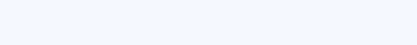But, since the high gradient factor is reached at the surface, HiGFDepth=0. So,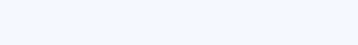Therefore, if you hit your first GF=0.3 at 110ft, then your LowGFDepth=110. Before you can ascend to 100ft you must let off enough TC pressure so that when you arrive at 100ft the GF of your TCs does not exceed 0.35 calculated as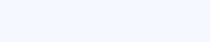You can ascend to 90ft when your TCs let off enough pressure at your 100ft stop so that when you reach 90ft your TC’s GF does not exceed 0.40 calculated as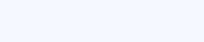The GF method allows you to ascend by walking that line all the way to the surface.

## Summary – Gradient Factors for Dummies

If you understood the above explanation, then you see why divers on RebreatherWorld say that setting your GF parameters to 10/90, or 10/80, etc. helps generate deep stops. The low GF of 10 means a stop must be generated when your TCs are only 10% of the way between ambient pressure and Buhlmann’s M-value, rather than 30% if you were to set the low GF to 30. Simply, the GF line just starts deeper.

The gradient factor method is a natural extension of Buhlmann’s tissue compartment model. Diver’s using computers implementing the gradient factor method should understand how modifying their GF parameters will alter their decompression profiles. My understanding is that it may be wise to consider altering your GF parameters based on dive characteristics, your physical condition, and your general attitude toward the risk of decompression illness. The gradient factor method provides the diver substantial flexibility in controlling their decompression profiles. Your responsibility is to choose the factors appropriate for you.

Safe diving!